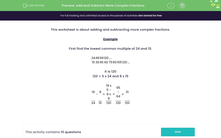# Add and Subtract Fractions with Different Denominators

In this worksheet, students will practise adding and subtracting more complex fractions.Key stage:  KS 3

Curriculum topic:   Number

Curriculum subtopic:   Use Four Operations for All Numbers

Popular topics:   Fractions worksheets, Percentages worksheets

Difficulty level:#### Worksheet Overview

Example

When adding or subtracting fractions we begin by finding the lowest common multiple (LCM) of both denominators.

Solve 15/24 - 8/15

First, find the LCM of the denominators which are 24 and 15.

 24 48 72 96 120 15 30 45 60 75 90 105 120 ...

The LCM is 120.

We multiplied 24 by 5 to get 120

and

We multiplied 15 by 8 to get 120

Ok, so now we know that the LCM of the denominators is 120, we need to turn our attention to the numerators in this sum.

We must multiply the numerator by the same number as we did by the denominator to find the LCM.

So, we multiply 15 by 5, and 8 by 8

 15 - 8 = 15 x 5 - 8 x 8 = 75 - 64 = 11 24 15 24 x 5 15 x 8 120 120

Answers in this activity should be given as reduced fractions.

Ready to give this a go?

### What is EdPlace?

We're your National Curriculum aligned online education content provider helping each child succeed in English, maths and science from year 1 to GCSE. With an EdPlace account you’ll be able to track and measure progress, helping each child achieve their best. We build confidence and attainment by personalising each child’s learning at a level that suits them.

Get started